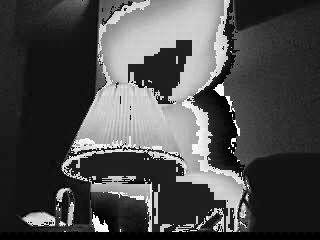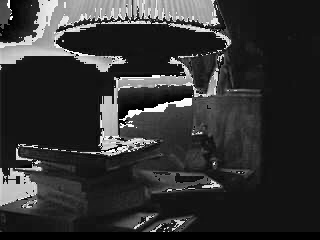# Screwy OpenCV Manipulations

I've been playing more with OpenCV and I think I'm missing something. I can't do any manipulations on the image data without really screwing it up. The only thing that doesn't seem to wash out the data out is moving pixels around without changing them. Not sure what I'm missing. Here's the few different manipulations and what they look like when they wash out.

Monochrome

```for(int i = 0; i < frame->height; i++) {
int offset = i*frame->width*3;
for(int j = 0; j < frame->width; j++) {
uchar temp = frame->imageData[offset+(j*3)]*0.114
+ frame->imageData[offset+(j*3)+1]*0.587
+ frame->imageData[offset+(j*3)+2]*0.299;
frame->imageData[offset+(j*3)] = temp;
frame->imageData[offset+(j*3)+1] = temp;
frame->imageData[offset+(j*3)+2] = temp;
}
}```Memory
This one just keeps five frames and then adds them in to create a faded composite, should be simple.

```if(0 == memory_frameCounter)
memory_frames = cvCloneImage(frame);
else if(2 == memory_frameCounter)
memory_frames = cvCloneImage(frame);
else if(4 == memory_frameCounter)
memory_frames = cvCloneImage(frame);
else if(6 == memory_frameCounter)
memory_frames = cvCloneImage(frame);
else if(8 == memory_frameCounter)
memory_frames = cvCloneImage(frame);
else if(10 <= memory_frameCounter)
memory_frameCounter = -1;

memory_frameCounter++;

for(int i = 0; i < frame->height; i++) {
for(int j =0; j < frame->width*3; j++) {
memory_agg = frame->imageData[(i*frame->width*3)+j];
for(int k = 0; k < 5; k++) {
memory_agg = (memory_agg + memory_frames[k]->imageData[(i*frame->width*3)+j])/2;
}
frame->imageData[(i*frame->width*3)+j] = memory_agg;
}
}```I just can't figure out what I'm doing wrong here.